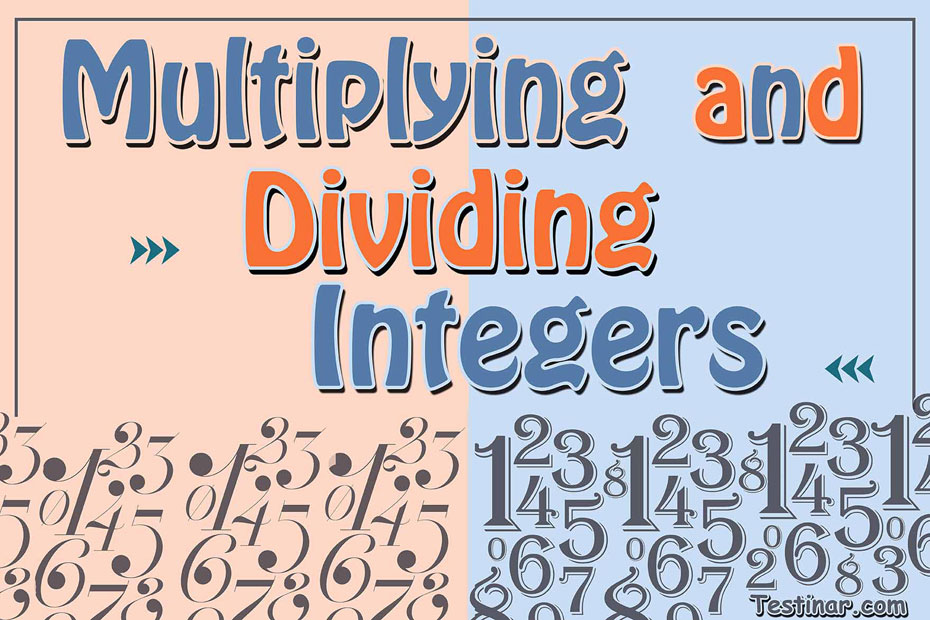## How to Multiply and Divide Integers

The term integers represent the meaning “intact” or “whole”. So, you can generally refer an integer as a whole number, except integers can be negative also!

What is an Integer?

So, to start, integers are part of the number system. You can plot them on a number line and an important thing to note is, integers can be negative as well as positive. For example: $$2 \ , \ 5 \ , \ 11 \ , \ 17 \ , \ -3 \ , \ -7 \ , \ -21,$$ etc. are all examples of integers.
Now, when we plot integers on the number line, there are two things to consider:

• Firstly, integers are plotted on the basis of their distance from the neutral number zero.
• Secondly, negative integers are plotted on the left side of zero, while positive ones are plotted on the right side. Also, the more negative an integer, the less will be its value.

Now, these considerations are important when we compare two or more integers or even perform mathematical operations with them. Remember: The multiplication of two integers with opposite signs will always give a negative integer (Same is the case with division). Also, the multiplication of two integers with the same sign will always give a positive integer (Same is the case with division).
So, now let’s look at the procedure to multiply or divide two integers.

How to Multiply and Divide Integers

To Multiply or Divide two integers, follow these steps:

• Firstly, perform general multiplication or division between the two integers and ignore their sign.
• Next, we have to decide the sign. So, if both signs are opposite, then we must always put a negative sign. Also, if both signs of the integers are same, we must use a positive sign.

Example:

• $$-12 \times +7 = -84$$
• $$-12 ÷ +6 = -2$$
• $$12 ÷ 3 = +4$$
• and so on.

### Exercises for Multiplying and Dividing Integers

1) $$(-6) \ \times \ (-3) \ \times \ (-10) \ =$$

2) $$1 \ \times \ (-9) \ \times \ (-10) \ =$$

3) $$24 \ \div \ (-4) \ =$$

4) $$(-4) \ \div \ (-2) \ =$$

5) $$2 \ \times \ (-5) \ =$$

6) $$(-7) \ \times \ (-6) \ =$$

7) $$(-4) \ \times \ 6 \ \times \ (-7) \ =$$

8) $$9 \ \times \ (-5) \ =$$

9) $$(-2) \ \times \ 1 \ \times \ (-4) \ =$$

10) $$3 \ \times \ 6 \ \times \ (-7) \ =$$

1) $$(-6) \ \times \ (-3) \ \times \ (-10) \ = \color{red}{-180}$$
Solution
Step 1: Ignore the signs and multiply the numbers.   $$6\ \times \ 3 \ \times \ 10 \ = 180$$
Step 2: Then determine the answer sign based on the rules: (negative) $$\times$$ (negative) $$=$$ positive and (positive) $$\times$$ (negative) $$=$$ negative, therefore the answer is: $$-180$$.
2) $$1 \ \times \ (-9) \ \times \ (-10) \ = \color{red}{90}$$
Solution
Step 1: Ignore the signs and multiply the numbers.   $$1\ \div \ 9 \ \div \ 10 \ = 90$$
Step 2: Then determine the answer sign based on the rules:(negative) $$\div$$ (positive) $$=$$ negative , and (negative) $$\times$$ (negative) $$=$$ positive
therefore the answer is: $$+ 90$$.
3)$$24 \ \div \ (-4) \ = \color{red}{-6}$$
Solution
Step 1: Ignore the signs and divide the numbers.  $$24 \ \div \ 4 = 6$$
Step 2: Then determine the answer sign based on the rules:(positive) $$\div$$ (negative) $$=$$ negative ,
therefore the answer is: $$- 6$$.
4)$$(-4) \ \div \ (-2) \ = \color{red}{2}$$
Solution
Step 1: Ignore the signs and divide the numbers.  $$4 \ \div \ 2 = 2$$
Step 2: Then determine the answer sign based on the rules:(negative) $$\div$$ (negative) $$=$$ positive,
therefore the answer is: $$- 2$$.
5)$$2 \ \times \ (-5) \ = \color{red}{-10}$$

6) $$(-7) \ \times \ (-6) \ = \color{red}{42}$$

7)$$(-4) \ \times \ 6 \ \times \ (-7) \ = \color{red}{168}$$

8) $$9 \ \times \ (-5) \ = \color{red}{-45}$$

9) $$(-2) \ \times \ 1 \ \times \ (-4) \ = \color{red}{8}$$

10) $$3 \ \times \ 6 \ \times \ (-7) \ = \color{red}{-126}$$

## Multiply and Divide Integers Practice Quiz

### AFOQT Math Full Study Guide

$25.99$13.99

### ParaPro Math in 10 Days

$17.99$12.99

### HSPT Math Study Guide 2020 - 2021

$20.99$15.99

### PSAT Math Full Study Guide

$25.99$13.99